Courses

# R.C. Mukherjee Test: Chemical Kinetics

## 30 Questions MCQ Test Chemistry for JEE | R.C. Mukherjee Test: Chemical Kinetics

Description
This mock test of R.C. Mukherjee Test: Chemical Kinetics for Class 12 helps you for every Class 12 entrance exam. This contains 30 Multiple Choice Questions for Class 12 R.C. Mukherjee Test: Chemical Kinetics (mcq) to study with solutions a complete question bank. The solved questions answers in this R.C. Mukherjee Test: Chemical Kinetics quiz give you a good mix of easy questions and tough questions. Class 12 students definitely take this R.C. Mukherjee Test: Chemical Kinetics exercise for a better result in the exam. You can find other R.C. Mukherjee Test: Chemical Kinetics extra questions, long questions & short questions for Class 12 on EduRev as well by searching above.
QUESTION: 1

Solution:

B + 3D = 2C + 4A

QUESTION: 2

### The rate constant for the forward reaction A(g)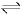2B(g) is 1.5 × 10-3 s-1 at 100 K. If 10-5 moles of A and 100 moles of B are present in a 10 litre vessel at equilibrium then rate constant for the backward reaction at this temperature is

Solution: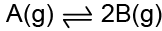Kf = = 1.5 × 10–3 s–1
At eq.m, Rf = Rb
Kf  [A] = Kb[B]2
On solving : Kb = 1.5 × 10-11

QUESTION: 3

### Reaction A + B → C + D follow's following rate law : rate = k[A]+1/2[B]1/2. Starting with initial conc. of 1 M of A and B each, what is the time taken for concentration of A of become 0.25 M. Given : k = 2.303 × 10-3 sec-1.

Solution:

Rate= K[A]1/2[B]1/2

Overall order of reaction is = 1/2+1/2=1

∴ It is a 1st order reaction.

For concentration of A to become 0.5M:-t1/2​=0.693​/k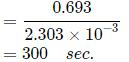It will require 2 half times for the concentration of A to become .0.25M.

∴ Time taken= 2×t1/2 sec

​=2×300

=600sec

QUESTION: 4

Consider the following first order competing reactions :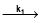A+ B      and y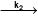C+D

if 50% of the reaction of X was completed when 96% of the reaction of Y was completed, the ratio of their rate constants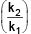is

Solution: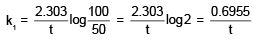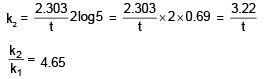QUESTION: 5

Units of rate constant for first and zero order reactions in terms of molarity (M) are respectively.

Solution:

sec–1, Msec–1

QUESTION: 6

The rate of a chemical reaction doubles for every 10°C rise of temperature. If the temperature is raised by 50°C, the rate of the reaction increases by about

Solution:

For every 10°C rise of temperature, the rate is doubled. Thus, the temperature coefficient of the reaction = 2
When temperature is increased by 50°, rate becomes
=2(50/10) = 25 times = 32 times

QUESTION: 7

For a reaction pA + qB → products, the rate law expression is r = k[A]l[B]m, then :

Solution:

pA + qB  → Products r = k[A]l[B]m
(p + q) may or may not be equal to (l + m) (order may or may not be equal to stoichiometric coefficient for a complex reaction.

QUESTION: 8

In the reaction : A + 2B → 3C + D, which of the following expression does not describe changes in the conc. of various species as a function of time :

Solution: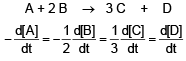QUESTION: 9

A first order reaction is 87.5% complete in an hour. The rate constant of the reaction is

Solution:

The correct answer is Option A.
KT  =   ln (a0 / at)
⇒ K*60 = ln (a0 / at
87.5 % completed in 1 hour.
Therefore, at = a0 - 0.875 a0
at = 0.125 a0
K * 60 = ln (a0 / 0.125 a0)
⇒ K * 60 = ln 8
⇒ K = 2.079 / 60
⇒ K = 0.0346 min-1

QUESTION: 10

Half-life period of a second order reaction is

Solution:

The correct answer is Option B.

Relation between half-life period of a reaction and initial concentration is as follows: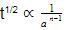For first order reaction         (Half life α a0 )
For second order reaction    (Half life ∝
1/a)
For third order reaction         (Half life ∝ 1a2)

QUESTION: 11

A first order reaction is 50% completed in 20 minutes at 27°C and in 5 min at 47°C. The energy of activation of the reaction is

Solution:

t1/2 = 20 min at 300 K
t’1/2 = 5 min at 320 K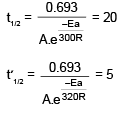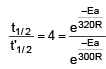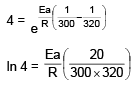On solving, Ea = 55303.12 J
= 55.3 KJ

QUESTION: 12

For the first order reaction A → B + C, carried out at 27°C if 3.8 × 10-16% of the reactant molecules exists in the activated state, the Ea (activation energy) of the reaction is

Solution:

A → B + C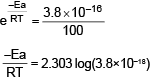Ea = 100 KJ/mol

QUESTION: 13

The rate constant, the activation energy and the Arrhenius parameter (A) of a chemical reaction at 25°C are 3.0 × 10-4 s-1, 104.4 kJ mol-1 and 6.0 × 10-4s-1respectively. The value of the rate constant at T → ∞ is

Solution:

At temperature = ∞
Rate constant = Arrhenious constant.

QUESTION: 14

The following mechanism has been proposed for the exothermic catalyzed complex reaction.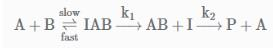If k1 is much smaller than k2. The most suitable qualitative plot of potential energy (P.E.) versus  reaction coordinate for the above reaction.

Solution: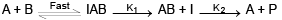Since
K1 <<< K2 = most Imp. peack will be higher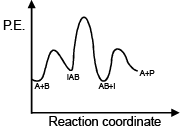QUESTION: 15

The activation energy of a reaction at a given temperature is found to be 2.303 RT J mol–1. The ratio of rate constant to the Arrhenius factor is

Solution:

The correct answer is option A
Arrhenius equation is,
rate constant, k=Ae−Ea​/RT
k=Ae−2.303RT/RT ⇒ k/A = e-2.303
On solving, we get
k/A = 10-1 or 0.1

QUESTION: 16

Consider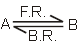+ heat, If activation energy for forward reaction is 100 kJ/mole then activation energy for backward reaction and heat of reaction is :

Solution: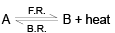Eaf = 100 KJ/mol
Eab = ? heat of reaction  = ?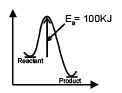Only one option because
Eb = Ea + ΔH.O. reaction

QUESTION: 17

In a reaction, the thershold energy is equal to :

Solution:

In a reaction, the threshold energy is equal to : Activation energy + Normal energy of reactants.

QUESTION: 18

The first order rate constant k is related to temperature as log k = 15.0 - (106/T). Which of the following pair of value is correct ?

Solution: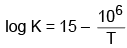log A = 15
⇒ A = 1015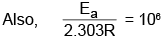Ea = 1.9 × 104  KJ

QUESTION: 19

When a graph between log K and 1/T is drawn a straight line is obtained. The temperature at which line cuts y-axis and x-axis.

Solution: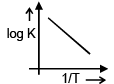When line cuts y axis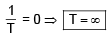When it cut  X -axis
log K = 0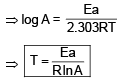QUESTION: 20

The rate constant, the activation energy and the frequency factor of a chemical reaction at 25°C are 3.0 × 10-2 s-1, 104.4 KJ mol-1 and 6.0 × 1014 s-1 respectively. The value of the rate constant as T → ∞ is :

Solution:

K = 3 × 10–2 s–1
Ea = 104.4 KJ/mol
A = 6 × 1014
Value of rate constant at T = ∞ will be equal to frequency factor  i.e. A = 6 × 1014 s-1

QUESTION: 21

The rate data for the net reaction at 25°C for the reaction X + 2Y → 3Z are given below :

[X0]   [Y0]   Time required for [Z] to increase by 0.005 mol per litre.

0.01   0.01   72 sec

0.02   0.005  36 sec

0.02   0.01    18 sec

The intial rate (as given by Z) is :

Solution: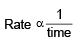Since,
Rate becomes four times by doubling the concentration of A
⇒ Order w.r.t  A is 2
Also,
by doubling the concentration of B, the rate becomes double
⇒ Order w.r.t. B is 1.

QUESTION: 22

The rate of production of NH3 in N2 + 3H2 → 2NH3 is 3.4 kg min-1. The rate of consumption of H2 is :

Solution: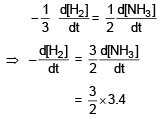= 5.1 Kgmin-1

QUESTION: 23

For a given reaction of first order it takes 20 min. for the conc. to drop from 1.0 M to 0.60 M. The time required for the conc. to drop from 0.60 M to 0.36 M will be :

Solution: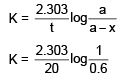Also,Since, Rate constant will be equal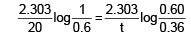On solving, t = 20 min

QUESTION: 24

For a first order reaction, the concentration of reactant :

Solution:

For a first order reaction, the concentration of the reactant varies exponentially with time (A = A0e–kt)

QUESTION: 25

Radioactivity of a sample (z = 22) decreases 90% after 10 years. What will be the half-life of the sample?

Solution:

Reaction is or zero order hence, Option C will be correct.

QUESTION: 26

Mathematical representation for t1/4 life for first order reaction is over is given by :

Solution:

t1/4 = time taken for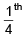decomposition.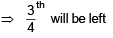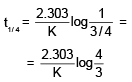QUESTION: 27

For a reaction A → Products, the conc. of reactant C0, aC0, a2C0, a3C0............ after time interval 0, t, 2t ............ where 'a' is constant. Then :

Solution:

If we calculate K, it comes constant every time i.e. reaction is of first order.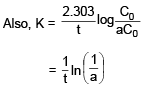QUESTION: 28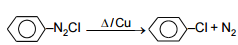Half-life is independent of conc. of A. After 10 minutes volume N2 gas is 10 L and after complete reaction 50 L. Hence rate constant in min-1 :

Solution: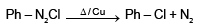Half life is independent of concentration
⇒ reaction is of first order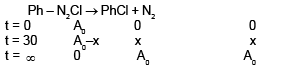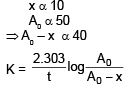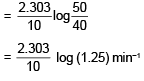QUESTION: 29

In a zero-order reaction for every 10° rise of temperature, the rate is doubled. If the temperature is increased from 10°C to 100°C, the rate of the reaction will become

Solution:

For rise in temperature, n=1
Therefore rate = 2n
= 21
= 2
When temperature is increased from 10o C to 100oC
change in temperature = 100 − 10 = 90o
therefore n=9 therefore, rate = 29
= 512 times.

QUESTION: 30

In acidic medium the rate of reaction between (BrO3)- & Br- ions is given by the expression, –[d(BrO3-) /dt] = K[BrO3-][Br-][H +]It means :

Solution: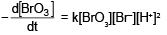D option is correct.